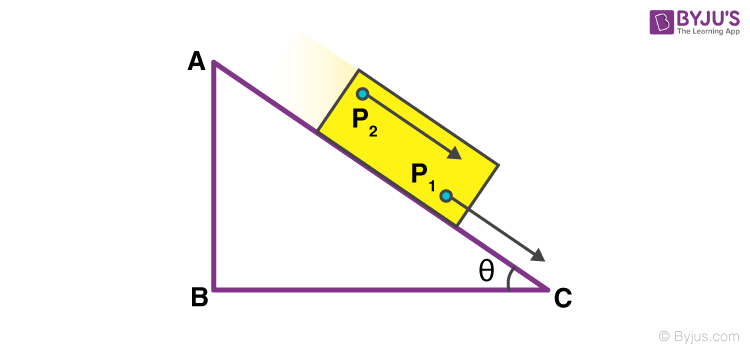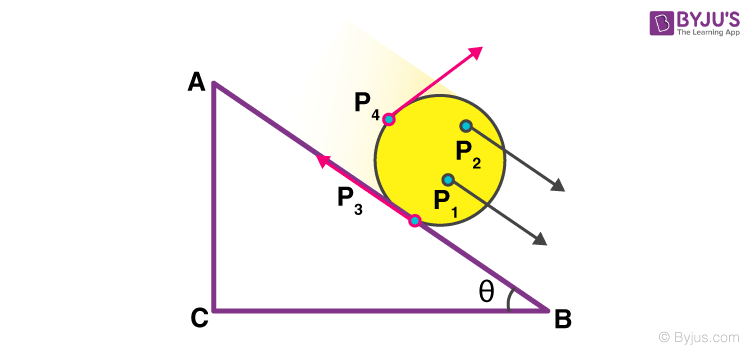# Rigid Bodies: Translational Motion And Rotational Motion

When a body does not change its position with respect to time, we say that the body is in rest. But, if a body changes its position with respect to time, we say that it is in motion. There are different types of motion: translational, rotational, periodic, and non-periodic. In this article, we will be discussing the translatory motion and rotational motion.

## What is Translatory Motion?

A type of motion in which all parts of the body move the same distance in a given time is known as the translatory motion. Translatory motion can be of two types: rectilinear and curvilinear.

If a body moves as a whole such that every part of the body moves through the same distance in a given time, then the body is said to be in translatory motion.

Given below in a table is the difference between rectilinear and curvilinear translatory motion, for your better understanding.

 Rectilinear Motion Curvilinear Motion When an object in translatory motion moves along a straight line, it is said to be in rectilinear motion When an object in translatory motion moves along a curved path, it is said to be in curvilinear motion A car moving along a straight path and the train moving in a straight track are examples of rectilinear motion A stone thrown up in the air at a certain angle and a car taking a turn are examples of curvilinear motion

### Translatory Motion Examples

Let us understand translational motion with the help of examples.

• Let’s imagine a rectangular block placed on the slanting edge of a right-angled triangle. If the block is assumed to slide down this edge without any side movement, every point in the rectangular block experiences the same displacement, and more importantly, the distance between the points is also maintained. In pure translational motion, every point in the body experiences the same velocity be it at any instant of time. Both the points, P1 and P2 undergo the exact same motions.A rectangular block sliding down the slanting edge of a right-angled triangle covers equal distance in equal intervals of time.
• A car moving in a straight line, the path of a bullet out of a gun, etc are examples of translational motion.

## Rotational Motion

Now let us imagine a circular block going down the edge of the right-angled triangle. Examining the location and orientation of different points on the cylindrical block will tell us something new. The points on the cylindrical body experience something much different from the rectangular block.A circular block rolling down the slanting edge of a right-angled triangle experiences different magnitude of velocity in different directions.

As shown by the arrows in the diagram representing the velocity, each point experiences a different magnitude of velocity in a different direction. Here the points are arranged with respect to an axis of rotation. Rotation is what you achieve when you constrain a body and fix it along a straight line. This means that the body can only turn around the line, which is defined as rotational motion. A ceiling fan, a potter’s wheel, a vehicle’s wheel are all examples of rotational motion.

Say you go to a bowling alley, and throw the bowling ball towards the pins. If you notice closely, you will see that the ball is not just moving forwards i.e. performing translational motion but it is also spinning on itself because of which you can spin and curve the entry of the ball; this motion is categorized as rotational motion. The motion of a rigid body which is not fixed or pivoted is either a pure translational motion or a combination of translational and rotational motion. Rigid bodies are fixed/pivoted experience motion which is rotational.

This is an article on the basics of motion in rigid bodies. Check out the center of mass calculations in rigid bodies in subsequent articles only on BYJU’S.

#### 1 Comment

1. sahil mathur

nice